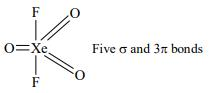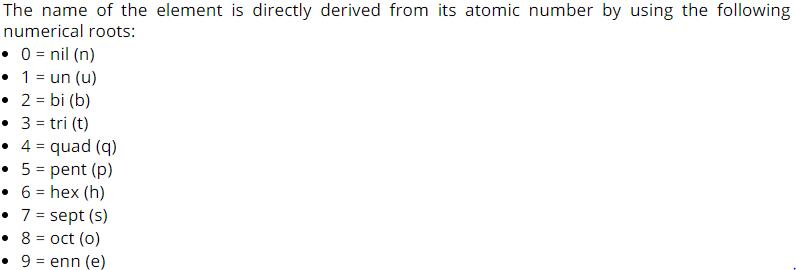Courses

# Test Level 1: Classification Of Elements & Periodicity In Properties

## 25 Questions MCQ Test Chemistry for JEE | Test Level 1: Classification Of Elements & Periodicity In Properties

Description
This mock test of Test Level 1: Classification Of Elements & Periodicity In Properties for JEE helps you for every JEE entrance exam. This contains 25 Multiple Choice Questions for JEE Test Level 1: Classification Of Elements & Periodicity In Properties (mcq) to study with solutions a complete question bank. The solved questions answers in this Test Level 1: Classification Of Elements & Periodicity In Properties quiz give you a good mix of easy questions and tough questions. JEE students definitely take this Test Level 1: Classification Of Elements & Periodicity In Properties exercise for a better result in the exam. You can find other Test Level 1: Classification Of Elements & Periodicity In Properties extra questions, long questions & short questions for JEE on EduRev as well by searching above.
QUESTION: 1

### f block of the Periodic Table consists of

Solution:

Lanthanides, Ce(Z = 58) ñ Lu(Z = 71) and Actinoids comprises of f block elements.

QUESTION: 2

### The elements charecterised by the filling of 4 f-orbitals, are:

Solution:

Elements characterized by the filling of 4f orbitals are lanthanides.

QUESTION: 3

### 14 elements after actinium is called

Solution:

The correct answer is Option B.

14 elements after actinium is called Actinides.

QUESTION: 4

An element belongs to Group 15 and third period of the periodic table. Its electronic configuration will be

Solution:

group is 15 so total valence electrons will be 5 while period is 3rd so n value of valence shell will be 3.

QUESTION: 5

It is now recognized that the ‘Modern Periodic Law’ is essentially the consequence of the

Solution:

Modern periodic law is essentially the result of periodic variation and electronic configuration.

QUESTION: 6

Metalloids show the properties of

Solution:

Metalloids shows both the properties of metals as well as non metals.

QUESTION: 7

One of the following options is not used for explaining atomic radius

Solution:

coordinate radius is not used for explaining atomic radius.

QUESTION: 8

Among the alkali metals cesium is the most reactive because

Solution:

Among the alkali metals cesium is the most reactive because the outermost electron is more loosely bound than the outermost electron of the other alkali metals.

QUESTION: 9

In which one of the given formulae of xenon compounds there are five α−bonds and three π-bonds in it?

Solution:QUESTION: 10

In the modern periodic table

Solution:

Horizontal rows are periods while vertical column are groups.

QUESTION: 11

Which of the following statements is false?

Solution:

Alkali metals always form ionic bonds.

QUESTION: 12

The order of Decreasing radius is

Solution:

vanderwaal radius > Metallic radius > Covalent radius.

QUESTION: 13

For alkali metals, which one of the following trends is INCORRECT?

Solution:

Actual trend of density is Li < K < Na < Rb

QUESTION: 14

Dmitri Mendeleev (1834-1907) and the German chemist, Lothar Meyer (1830-1895) proposed arranging elements in

Solution:

They arranged elements in increasing order of atomic weights.

QUESTION: 15

According to the recommendation of International Union of Pure and Applied Chemistry (IUPAC), the groups in the modern periodic table are numbered from

Solution:

they are numbered from 1 to 18 1 is alkali metal group and 18 is noble gases.

QUESTION: 16

Ionization enthalpy increases across a period because

Solution:

as we move from left to right in a period atomic radii decreases and due to effective nuclear charge outermost electrons are held more closely to the nucleus.

QUESTION: 17

Choose one of the following in th increasing order of bond length

Solution:

As the size increases bond length also increases.

QUESTION: 18

For the four successive transition elements (Cr, Mn, Fe and Co), the stability of + 2 oxidation state will be there in which of the following order? (At. nos. Cr = 24, Mn = 25, Fe = 26, Co = 27)

Solution:

Mn has most stable +2 stable because Mn+2 had d5 configuration which is most stable.

QUESTION: 19

A transition metal ion exists in its highest oxidation state. It is expected to behave as

Solution:

When a transition metal ion is in highest oxidation state it tends to lower its oxidation state by gaining electrons. thus it itself reduces and oxidizes the substance from which it accepts electron. So, it acts as oxidising agent.

QUESTION: 20

The sequence of ionic mobility in aqueous solution is

Solution:

Smaller the size of cation, higher will be the hydration and its effective size will increase and hence mobility in aqueous solution will decrease. Larger size ions have more ionic mobility due to less hydration. Thus the degree of hydration of M+ ions decreases from Li+ to Cs+. Consequently the radii of the hydrated ion decreases from Li+ to Cs+. Hence the ionic conductance of these hydrated ions increases from Li+ to Cs+

QUESTION: 21

In the periodic table Electronegativity generally

Solution:

electronegativity increases on moving from left to right while decreases as we move down the group.

QUESTION: 22

Al(3+)<Mg(2+)<Na+<F−<O(2−)<N(3−) The above can be aptly described as

Solution:

They are isoelectronic species with same number of electrons. Higher is the atomic number, higher will be the effective nuclear charge, lower will be the size.

QUESTION: 23

Generally, the first ionization energy increases along a period. But there are some exceptions. One which is NOT an exception is ______.

Solution:

Na and Mg are not an exception.

QUESTION: 24

Which of the following will have the most negative electron gain enthalpy and which one the least negative? P, S, Cl, F.

Solution:

Cl just need one more electrons to get fully filled electronic configuration so it has most negative electron gain enthalpy while P has d3 configuration which is already stable so it cannot accept one electron extra easily so have large positive electron gain enthalpy.

QUESTION: 25

For Atomic numbers greater than 100 IUPAC has made recommendation that until a new element ‘s discovery is proved, and its name is officially recognized, a systematic nomenclature be derived directly from the atomic number of the element using the numerical roots for 0 and numbers 1-9. The name 'sept' in this scheme corresponds to the digit

Solution:

sept is for 7.